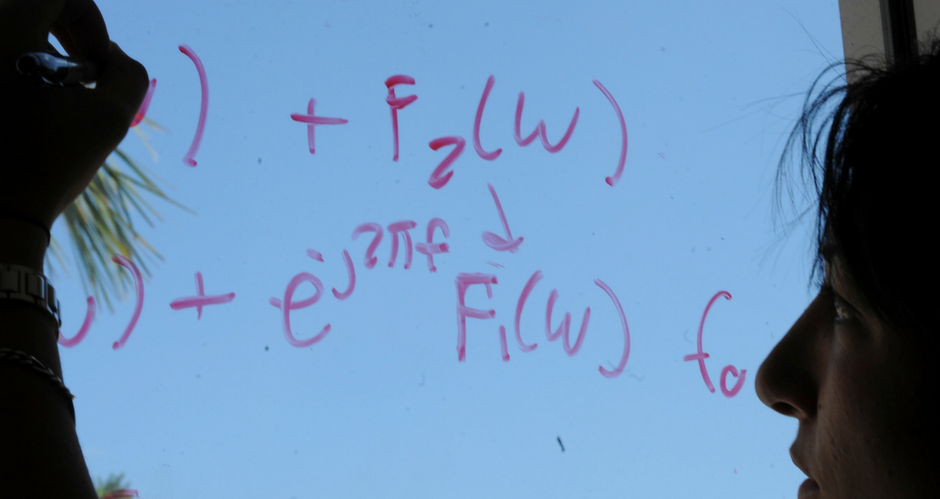## Mathematics and System Engineering Faculty Publications

### The Kolmogorov equation with time-measurable coefficients

Article

#### Publication Title

Electronic Journal of Differential Equations

#### Abstract

Using both probabilistic and classical analytic techniques, we investigate the parabolic Kolmogorov equation $$L_t v +\frac {\partial v}{\partial t}\equiv \frac 12 a^{ij}(t)v_{x^ix^j} +b^i(t) v_{x^i} -c(t) v+ f(t) +\frac {\partial v}{\partial t}=0$$ in $H_T:=(0,T) \times E_d$ and its solutions when the coefficients are bounded Borel measurable functions of $t$. We show that the probabilistic solution $v(t,x)$ defined in $\bar H_T$, is twice differentiable with respect to $x$, continuously in $(t,x)$, once differentiable with respect to $t$, a.e. $t \in [0,T)$ and satisfies the Kolmogorov equation $L_t v +\frac {\partial v}{\partial t}=0$ a.e. in $\bar H_T$. Our main tool will be the Aleksandrov-Busemann-Feller Theorem. We also examine the probabilistic solution to the fully nonlinear Bellman equation with time-measurable coefficients in the simple case $b\equiv 0,\,c\equiv 0$. We show that when the terminal data function is a paraboloid, the payoff function has a particularly simple form.

1

14

7-13-2003

COinS